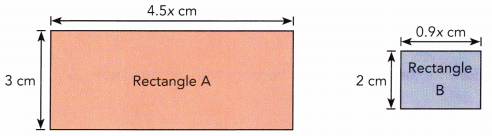# Math in Focus Grade 7 Chapter 3 Lesson 3.2 Answer Key Subtracting Algebraic Terms

Go through the Math in Focus Grade 7 Workbook Answer Key Chapter 3 Lesson 3.2 Subtracting Algebraic Terms to finish your assignments.

## Math in Focus Grade 7 Course 2 A Chapter 3 Lesson 3.2 Answer Key Subtracting Algebraic Terms

### Math in Focus Grade 7 Chapter 3 Lesson 3.2 Guided Practice Answer Key

Copy and complete to simplify each expression.

Question 1.
0.7y – 0.4y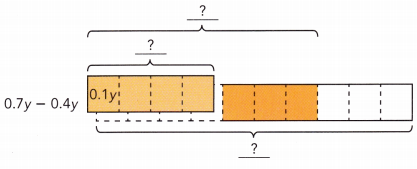0.7y – 0.4y =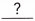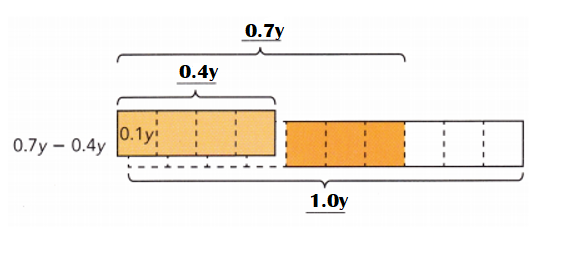Explanation:
0.7y – 0.4y
= 0.3y.

Question 2.
1.1a – 0.2a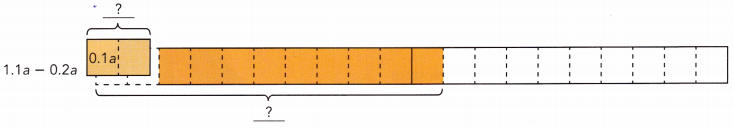1.1a – 0.2a =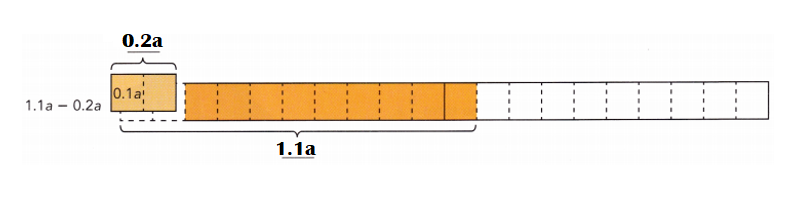Explanation:
1.1a – 0.2a
=0.9a

Question 3.
1.2y – y
1.2y – y =1.2y – y = 0.2y.

Explanation:
1.2y – y
= 0.2y.

Copy and complete to simplify each expression.
Question 4.
$$\frac{4}{9}$$x – $$\frac{1}{3}$$x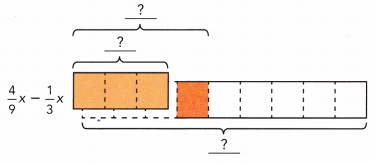The LCD of $$\frac{4}{9}$$ and $$\frac{1}{3}$$ is.
So, x is divided intox sections.
$$\frac{4}{9}$$x – $$\frac{1}{3}$$x =$$\frac{4}{9}$$x – $$\frac{1}{3}$$x = $$\frac{1}{9}$$x.

Explanation:
The LCD of $$\frac{4}{9}$$ and $$\frac{1}{3}$$ is 9.
So, x is divided into 9  x sections.
$$\frac{4}{9}$$x – $$\frac{1}{3}$$x
= [4 – (1 × 3)]x ÷ 9
= (4 – 3)x ÷ 9
= x ÷ 9 or $$\frac{1}{9}$$x

Question 5.
$$\frac{3}{4}$$p – $$\frac{1}{6}$$p
$$\frac{3}{4}$$p – $$\frac{1}{6}$$p ==Rewrite the coefficients as fractions with denominator.
$$\frac{3}{4}$$p – $$\frac{1}{6}$$p = $$\frac{7}{12}$$p.

Explanation:
$$\frac{3}{4}$$p – $$\frac{1}{6}$$p
LCD of 4 n 6 = 12.
= [(3 × 3) – (1 × 2)]p ÷ 12
= (9 – 2)p ÷ 12
= 7p ÷ 12 or $$\frac{7}{12}$$p

### Math in Focus Course 2A Practice 3.2 Answer Key

Simplify each expression with decimal coefficients.
Question 1.
0.8y – 0.7y
0.8y – 0.7y = 0.1y.

Explanation:
0.8y – 0.7y = 0.1y.

Question 2.
0.9x – 0.6x
0.9x – 0.6x = 0.3x.

Explanation:
0.9x – 0.6x = 0.3x.

Question 3.
1.7p – 0.4p
1.7p – 0.4p = 1.3p.

Explanation:
1.7p – 0.4p = 1.3p.

Question 4.
1.9h – 0.9h
1.9h – 0.9h = 1.0h.

Explanation:
1.9h – 0.9h = 1.0h.

Question 5.
1.3m – 0.5m
1.3m – 0.5m = 0.8m.

Explanation:
1.3m – 0.5m = 0.8m.

Question 6.
1.6n – 0.8n
1.6n – 0.8n = 0.8n.

Explanation:
1.6n – 0.8n = 0.8n.

Simplify each expression with fractional coefficients.
Question 7.
$$\frac{5}{6}$$x – $$\frac{1}{6}$$x
$$\frac{5}{6}$$x – $$\frac{1}{6}$$x = $$\frac{2}{3}$$x

Explanation:
$$\frac{5}{6}$$x – $$\frac{1}{6}$$x
= (5 – 1)x ÷ 6
= 4x ÷ 6
= 2x ÷ 3 or $$\frac{2}{3}$$x

Question 8.
$$\frac{7}{8}$$x – $$\frac{5}{8}$$x
$$\frac{7}{8}$$x – $$\frac{5}{8}$$x = $$\frac{1}{4}$$x.

Explanation:
$$\frac{7}{8}$$x – $$\frac{5}{8}$$x
= (7 – 5)x ÷ 8
= 2x ÷ 8
= x ÷ 4 or $$\frac{1}{4}$$x

Question 9.
$$\frac{9}{5}$$x – $$\frac{1}{5}$$x
$$\frac{9}{5}$$x – $$\frac{1}{5}$$x = $$\frac{8}{5}$$x.

Explanation:
$$\frac{9}{5}$$x – $$\frac{1}{5}$$x
= (9 – 1)x ÷ 5
= 8x ÷ 5 or $$\frac{8}{5}$$x.

Question 10.
$$\frac{8}{3}$$p – $$\frac{1}{3}$$p
$$\frac{8}{3}$$p – $$\frac{1}{3}$$p = $$\frac{7}{3}$$p.

Explanation:
$$\frac{8}{3}$$p – $$\frac{1}{3}$$p
= (8 – 1)p ÷ 3
= 7p ÷ 3 or $$\frac{7}{3}$$p.

Simplify each expression with fractional coefficients by rewriting the fractions.
Question 11.
$$\frac{1}{4}$$a – $$\frac{1}{8}$$a
$$\frac{1}{4}$$a – $$\frac{1}{8}$$a = $$\frac{1}{8}$$a.

Explanation:
$$\frac{1}{4}$$a – $$\frac{1}{8}$$a
= LCD of 4 n 8 = 8.
= (2 – 1)a ÷ 8
= a ÷ 8 or $$\frac{1}{8}$$a

Question 12.
$$\frac{5}{6}$$m – $$\frac{2}{3}$$m
$$\frac{5}{6}$$m – $$\frac{2}{3}$$m = $$\frac{1}{6}$$m

Explanation:
$$\frac{5}{6}$$m – $$\frac{2}{3}$$m
= LCD of 6 n 3 = 6.
= [5 – (2 × 2)]m ÷ 6
= (5 – 4)m ÷ 6
= m ÷ 6 or $$\frac{1}{6}$$m

Question 13.
$$\frac{5}{3}$$b – $$\frac{1}{6}$$b
$$\frac{5}{3}$$b – $$\frac{1}{6}$$b = $$\frac{1}{2}$$b.

Explanation:
$$\frac{5}{3}$$b – $$\frac{1}{6}$$b
= LCD of 3 n 6 = 6.
= [(5× 2) – 1]b ÷ 6
= (10 – 1)b ÷ 6
= 9b ÷ 6
= 3b ÷ 6
= b ÷ 2 or $$\frac{1}{2}$$b.

Question 14.
$$\frac{7}{4}$$x – $$\frac{1}{8}$$x
$$\frac{7}{4}$$x – $$\frac{1}{8}$$x = $$\frac{13}{8}$$x.

Explanation:
$$\frac{7}{4}$$x – $$\frac{1}{8}$$x
LCD of 4 n 8 = 8.
= [(7 × 2) – 1]x ÷ 8
= (14 – 1)x ÷ 8
= 13x ÷ 8 or $$\frac{13}{8}$$x.

Question 15.
$$\frac{4}{5}$$p – $$\frac{1}{3}$$p
$$\frac{4}{5}$$p – $$\frac{1}{3}$$p = $$\frac{2}{3}$$p.

Explanation:
$$\frac{4}{5}$$p – $$\frac{1}{3}$$p
LCD of 5 n 3 = 15.
= [(4 × 3) – (1 × 5)]p ÷ 15
= (15 – 5)p ÷ 15
= 10p ÷ 15
= 2p ÷ 3 or $$\frac{2}{3}$$p.

Question 16.
$$\frac{3}{4}$$r – $$\frac{2}{3}$$r
$$\frac{3}{4}$$r – $$\frac{2}{3}$$r = $$\frac{1}{12}$$r.

Explanation:
$$\frac{3}{4}$$r – $$\frac{2}{3}$$r
LCD of 4 n 3 = 12.
= [(3 × 3) – (2 × 4)]r ÷ 12
= (9 – 8)r ÷ 12
= r ÷ 12 or $$\frac{1}{12}$$r.

Question 17.
$$\frac{11}{7}$$k – $$\frac{1}{2}$$k
$$\frac{11}{7}$$k – $$\frac{1}{2}$$k = $$\frac{15}{14}$$k.

Explanation:
$$\frac{11}{7}$$k – $$\frac{1}{2}$$k
LCD of 7 n 2 = 14.
= [(11 × 2) – (1 × 7)]k ÷ 14
= (22 – 7)k ÷ 14
= 15k ÷ 14 or $$\frac{15}{14}$$k.

Question 18.
$$\frac{7}{4}$$d – $$\frac{3}{5}$$d
$$\frac{7}{4}$$d – $$\frac{3}{5}$$d = $$\frac{23}{20}$$d.

Explanation:
$$\frac{7}{4}$$d – $$\frac{3}{5}$$d
LCD of 4 n 5 = 20.
= [(7 × 5) – (3 × 4)]d ÷ 20
= (35 – 12)d ÷ 20
= 23d ÷ 20 or $$\frac{23}{20}$$d.

Question 19.
Math Journal Matthew simplified the algebraic expression $$\frac{3}{2}$$x – $$\frac{1}{3}$$x as shown below.
$$\frac{3}{2}$$x – $$\frac{1}{4}$$x = $$\frac{18}{12}$$x – $$\frac{4}{12}$$x
= $$\frac{14}{12}$$x
Is Matthew’s simplification correct? Why or why not?
Matthew’s simplification is incorrect because $$\frac{3}{2}$$x – $$\frac{1}{4}$$x  is not equal to $$\frac{18}{12}$$x – $$\frac{4}{12}$$x.

Explanation:
$$\frac{3}{2}$$x – $$\frac{1}{4}$$x
LCD of 2 n 4 = 4.
= [(3 × 2) – (1 × 1)]x ÷ 4
= (6 – 1)x ÷ 4
= 5x ÷ 4 or $$\frac{5}{4}$$x.

$$\frac{18}{12}$$x – $$\frac{4}{12}$$x
= (18 – 4)x ÷ 12
= 14x ÷ 12
= 7x ÷ 6 or $$\frac{7}{6}$$x.

$$\frac{14}{12}$$x = $$\frac{7}{6}$$x

Question 20.
Rectangle A, shown below, is larger than rectangle B. Write and simplify an algebraic expression that represents the difference in the areas of the two rectangles.